## Beginners Guide to Top 4 Regression Techniques in Data Science

Research in data science is incomplete with performing regression analysis. Running a regression test is no-brainer, provided you know ‘A-Z’ about the types & features of regression analysis techniques. While a handful of scholars are aware of this concept, to many others, it still remains a baffling topic. In this article, let’s have a detailed look at the popular types of regression analysis techniques.

Regression analysis, a parametric statistical technique is used for determining the relationships between the criterion variable or the dependent variables with the one or more independent variables. It enables the researcher to determine the changes in the independent variable as a result of dependent variables. The added advantage of using regression analysis include trend forecasting, determination of the impact of the predictors, and forecasting the effect. Additionally, it also helps to determine the change in the independent variable due to the conditional expectation of dependent variables.

Typically, regression analysis employs a linear function to appropriate the dependent variable. The general equation of regression analysis is given by Y = ?o + ?1X + ?, where ‘1’ is the slope, ‘?’ is the error, ‘o’ is the intercept, ‘X’ is the independent variable, and ‘Y’ is the dependent variable.

Of late, regression techniques are considered as a working horse in data science as it can be used to test the hypothesis, determine the strength of predictors, and many more.

Some of the most popular regression techniques used in data science include:

• Linear regression

• Ridge regression

• Logistic regression

• Lasso regression

1. Linear regression

Linear regression, regarded as a modeling technique, is primarily used in predictive analysis. It is a linear approach employed for modeling the relationship between the dependent variable & multiple predictors or independent variable. It mainly focuses on the conditional probability of the responses. Here, the dependent variable is continuous, whereas the independent variable can be continuous or discrete. This type of regression establishes a relationship independent (X) and dependent variable (Y) using the best fit straight line and is represented by an equation,

Y=a+b*X + e, where ‘a’ is the intercept, ‘b’ is the slope and ‘e’ is the error.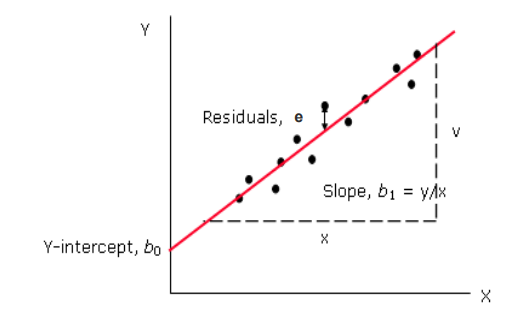Some of the important points to consider while using this technique include: linear regression is sensitive to outliers, and there must be a linear relationship dependent & independent variables.

2. Logistics regressions

Logistic regression is a foremost regression analysis technique utilised to predict a categorical variable. It is a case of  generalised linear model (GLM) models and is widely used in data science to describe the data and explain the relationship between dependent binary variable & one or more nominal/ordinal or ratio-level independent variable.

The logistics regression follows an S-shaped graph and is defined by an equation,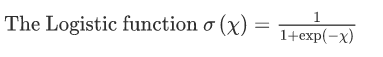and the logit transformation is defined by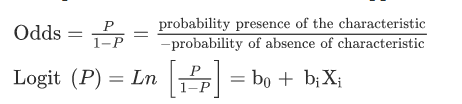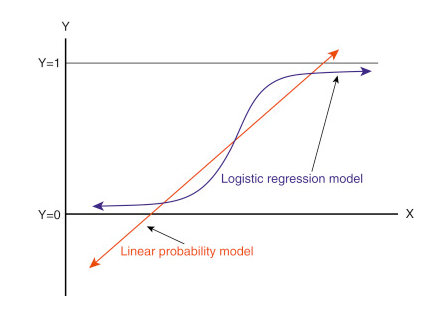Where P : the probability of the event

The value can be calculated using the inverse :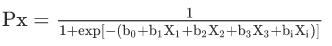Unlike linear regression, logistic regression doesn’t demand a linear relationship between the dependent & independent variables. However, it requires large sample sizes.

3. Lasso regression

Lasso abbreviated as Least Absolute Shrinkage and Selection Operator is a type of regression analysis technique that includes data shrinkage. This regression uses quadratic programming and is particularly applied when models exhibit a high level of correlation (multicollinearity). The benefit of using this technique is it minimises the variability and enhances the accuracy of linear regression models.

The equation for Lasso regression is given by,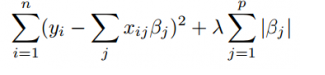Where λ is the shrinkage factor.

Case1:  When λ = 0, no parameters are eliminated. It is similar to linear regression.

Case 2: With the increase in λ, the biases of the sample increases. It also set all the coefficients to zero and then they are eliminated to make it more interpretable.

Case 3: When the λ decreases, the variance increases.

4. Ridge regression

Ridge regression analysis is used only if the data suffers multicollinearity. It helps in analysing the data that has a greater number of predictors than observations. Due to the higher number of predictors in the data, the number of variance in the data can be larger than the actual value, which results in unbiasing of least squares estimates. The ridge regression reduces the standard error by adding the degree of bias so that the net effect will give estimates that would be more accurate. This type of regression uses shrinkage estimator as ridge estimator. This estimator is then used to enhance the least square estimates.

The ridge regression can be written in matrix form :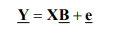Where  ‘Y’ is the dependent variable

‘ X’ is the independent variable

‘B’ is the regression coefficients to be estimated

‘e’ errors are residuals

Unlike Lasso regression, Ridge technique shrinks the data but never reaches 0 value.

Regression analysis techniques can work wonders which chosen as per the requirement of the study. Determine the type of collected data, select the best-fit technique and accomplish the data analysis process.

Category : Research